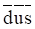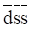Chapter 12, Problem 24P

Chapter
Section
Textbook Problem

. Distinguish a particle composed of a combination ofquarks from one composed of dus quarks in as many ways as you can. Do the same for a dss combination and acombination.

To determine

To distinguish a particle made up of d¯u¯s¯ and dus. And similarly, for the particle made up of d¯s¯s¯ and dss.

Explanation

Introduction:

The baryon is a type of composite subatomic particle which consists of quarks and antiquarks.

The subatomic particles in baryon category are made up of up, down and strange quark.

Compare the particle composed of d¯u¯s¯ and dus.

• The baryon number of both combinations is different:

Baryon number for each quark is +13 and for each anti quark is 13.

So, Baryon number for d¯u¯s¯ combination is 131313=1

And Baryon number for dsu combination is +13+13+13=+1

• The strangeness of both combinations is different:

Strangeness number for up, down, antiup and antidown quark is 0 and for strange quark is 1 and for anti-strange quark is +1

So, strangeness number for d¯u¯s¯ combination is 0+0+1=+1

And strangeness number for dsu combination is 0+01=1

Compare the particle composed of d¯s¯s¯ and dss.

• The baryon number of both combinations is different:

Baryon number for each quark is +13 and for each anti quark is 13

Still sussing out bartleby?

Check out a sample textbook solution.

See a sample solution

The Solution to Your Study Problems

Bartleby provides explanations to thousands of textbook problems written by our experts, many with advanced degrees!

Get Started

Find more solutions based on key concepts